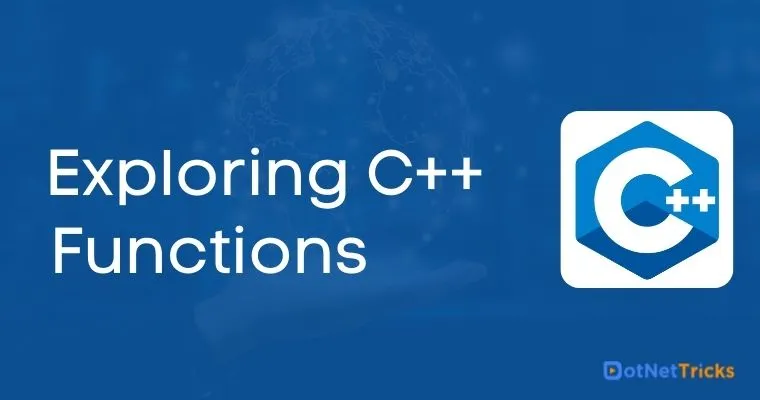# Exploring C++ Functions

22 Aug 2022
Intermediate
6.41K Views

Functions are a logical grouping of statements that perform a specific task. By adding a function, you can avoid writing the same piece of code repeatedly for different input values within the program. You just need to call the function to perform the operation. Functions help in achieving a structured way of programming.

### Syntax of a C++ function

```return type function_name (function parameters)
{
//function body;
….
}
```

Return Type : This suggests what type of value the function will return as output. The return types can be integer, character, etc., Sometimes, a function need not have to return a value. In this case, you can define the function with the return type as void.

Function Name : The name of the function. E.g., foo. You can use this name to call the function from anywhere within the scope of the program.

Function Parameters : You can define variables that hold the argument values that are passed when the function is called.

#### Example of a function declaration in C++ programming language

``` #include <iostream>
using namespace std;

int mult (int a, int b)
{
int prod;
prod = a * b;
cout << "The product is : " << prod << '\n';
}

int main()
{
int x, y;
cout << "Enter the value of x & y : " << '\n';
cin >> x >> y;
mult (x, y);
}
```

The result of this program will be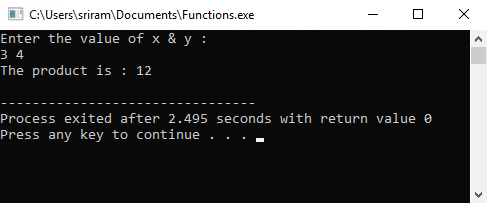## Types of Functions in C++

There are two types of functions in C++

1. Library (Built-in) Functions

2. User-defined Functions

### Library Functions

Library functions or Built-in functions are functions that are already available within the C++ libraries. You can make use of these functions within the program. For example, if you want to calculate the square root of a number or calculate the exponential of a number, these functions are found within the cmath header file. Therefore, it is important to include the header file in the program.

Example Program for C++ Library Functions
``` #include <iostream>
#include <cmath>
using namespace std;

int main()
{
double x, y;
cout << "Enter the value : " << '\n';
cin >> x;
y=exp (x);
cout << "The value of y is : " << y << '\n';
}
```

The output of this program will be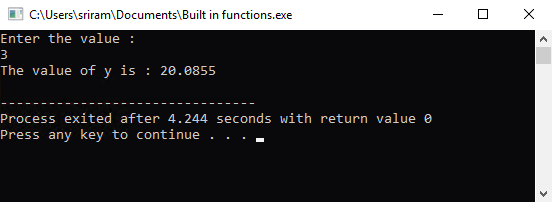### User-defined Functions

User defined functions are functions are that defined by the users to perform an operation. The user defined function can be accessed from anywhere within the program. The syntax of user-defined functions is :

```
#include<iostream>

int userdefinedfunctionname()
{
//function statements;
}

int main()
{
userdefinedfunctionname();
}
```

When you run the program, the main() function will be called first and the statements within the main function will be executed. As a part of the main program, the user-defined function will be called from the main function. The scope of the program will now shift from the main function to the user-defined function. All the statements within the user-defined function will be executed and then the scope of the function will return back to the main function where the statements after the user-defined function call are executed.

Example Program
```
#include <iostream>
using namespace std;

int sum (int x, int y)
{
int z;
z = x + y;
cout << "The sum is : " << z << '\n';
}

int main()
{
int value1, value2;
cout << "Enter the value of value1 & value2 : " << '\n';
cin >> value1 >> value2;
sum (value1, value2);
}
```

The output of this program will be :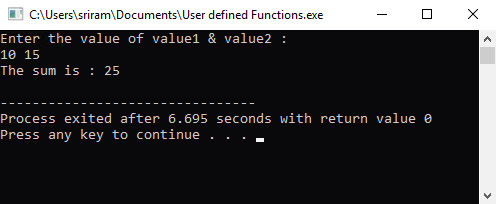## Function Parameters

In C++, a parameter is something like a placeholder. When you call the function, you must pass values to the parameter (if the function contains defined parameters). Defining parameters to functions is completely optional. In the previous example, the function ‘sum’ takes two parameters x and y as input. When we called the ‘sum’ function from the main program, we passed the values value1 and value2 to the function as the input. When you call a function that contains arguments, you can call the function in two different ways :

• Call by Value

• Call by Reference

### Call by Value

When a function is called by value, only the actual value of the argument is passed into the parameter of the function. In simple terms, the called function will create a copy of the arguments that are passed into it from the calling function. Both the parameter types are stored in different memory locations. Let’s take an example to understand the call by value concept.

```#include <iostream>
using namespace std;

int func (int x)
{
x++;
cout << "The value within the called function is : " << x << '\n';
}

int main()
{
int value1=10;
cout << "The value within the main functions is : " << value1 << '\n';
func (value1);
}
```

The output of this program will be :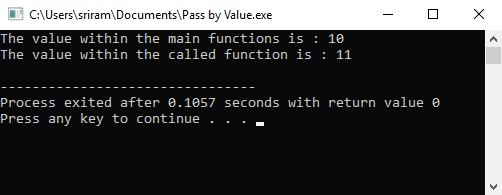As you can observe, the value in the original variable value1 is not changed since the value of the variable is passed as a value. Therefore, a copy of the value is passed into the function which gets incremented by 1 within the function. Once the program exits the function, the value gets destroyed from the memory. Therefore, within the main() function, the value of value1 will be 10.

### Call by Reference

When a function is called by reference, the reference of the argument is passed into the parameter of the function. The function will receive the argument and store it within the same memory address. Let’s take an example to understand the call by reference concept.

```#include <iostream>
using namespace std;

void f1(int *x, int *y)
{
int temp;
temp = *x;
*x = *y;
*y = temp;
}

int main()
{
int a=1, b=2;
cout << "The value of a & b before interchange is : " << a << b << '\n';
f1(&a, &b);
cout << "The value of a & b after interchange is : " << a << b << '\n';
}
```

The output of this program will be :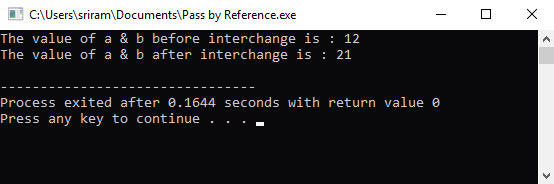In this example, the address of a and b is passed from the main function (the calling function) to the function f1 (the called function).In the f1 function, we perform an operation to swap the values of a and b. Since there is no separate memory allocated, the value will get swapped and the scope of the program will come back to the main function.

##### Summary

In this chapter, we have covered the following topics :

• Introduction to Functions n C++

• Types of functions

• Function Parameters

Learn to Crack Your Technical Interview